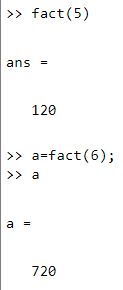# User defined function in MATLAB

• Difficulty Level : Medium
• Last Updated : 20 Aug, 2020

Functions let you do a specific task. User defined functions are the functions created by the users according to their needs. This article explains how the user defined function in MATLAB is created.

Syntax : function [a1,…,an] = func(x1,…,xm)
func is the function name
a1,…,an are outputs
x1,…,xm are inputs
Function name is required, whereas input and output arguments are optional.

For making a user defined function in MATLAB, go to Home -> New -> Function. The function template appears as-

```function [outputArg1,outputArg2] = untitled(inputArg1,inputArg2)
% UNTITLED Summary of this function goes here
% Detailed explanation goes here
outputArg1 = inputArg1;
outputArg2 = inputArg2;
end```

Change the function name and save the file with the changes made. Save the file either in the current folder or in a folder on the MATLAB search path. The name of the function and the file should be same. For example, to create a function for calculating factorial, the code is:

 `function` `f = fact(n)``    ``f = 1;``    ``i = 1;``    ``while` `i <= n``        ``f = f * i;``        ``i = i + 1;``    ``end``end`

Save it and it can be run from the command window. The value of the fact() function returned, can also be stored in a variable. Code output for different values of n being passed :My Personal Notes arrow_drop_up